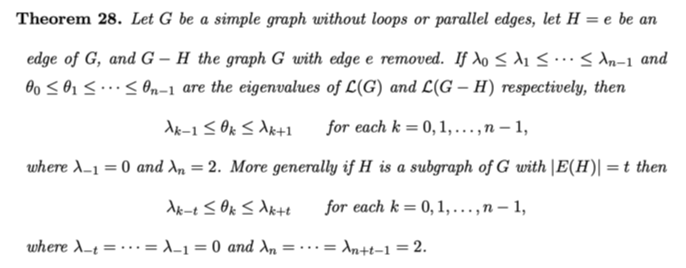# What happens to eigenvalues when edges are removed?

I am stuck at the following :

Let $$G$$ be a graph and $$A$$ is its adjacency matrix. Let the eigenvalues of $$A$$ be $$\lambda_1\le \lambda_2\leq \cdots \leq \lambda_n$$.

If we remove some edges from the graph $$G$$ and form the graph $$H$$ keeping the number of vertices same, is there any result how the smallest eigenvalue of $$H$$ is related to the smallest eigenvalue of $$G$$?

I know Cauchy Interlacing Theorem which gives the relation between eigenvalues of a graph and its induced subgraph when some vertices are removed.

I want to know what happens when edges are removed keeping the number of vertices same. Can someone help please?

The question stands as:

Let $$G$$ be a graph and $$A_G$$ is its adjacency matrix. Let the eigenvalues of $$A_G$$ be $$\lambda_1\le \lambda_2\leq \cdots \leq \lambda_n$$.

Let $$H$$ be a subgraph of $$G$$ which has $$n$$ vertices as $$G$$ but some edges have been removed from $$G$$ to form $$H$$.$$A_H$$ is its adjacency matrix.

Let the eigenvalues of $$A_H$$ be $$\mu_1\le \mu_2\leq \cdots \leq \mu_n$$.

Is $$\mu_1\ge \lambda_1$$ or $$\mu_1\le \lambda_1$$?

If someone can give any reference like book or paper , I will be grateful.

The smallest eigenvalue can go up or down when an edge is removed.

For "down": $$G=K_n$$ for $$n\ge 3$$.

For "up": Take $$K_n$$ for $$n\ge 1$$ and append a new vertex attached to a single vertex of the original $$n$$ vertices. Now removing the new edge makes the smallest eigenvalue go up.

Both of these follow from the fact that the smallest eigenvalue of a connected graph with $$n\ge 2$$ vertices is $$\le -1$$ with equality iff the graph is complete.

It has something to do with whether the two corresponding eigenvector entries have the same or opposite sign, but I don't know if that relationship can be made precise.

Here's a statement from the book "Spectra of Graphs" by Brouwer and Haemers concerning the largest eigenvalue of the adjacency matrix. It implies that $$\lambda_n \geq \mu_n$$.

Proposition 3.1.1 The value of the largest eigenvalue of a graph does not increase when vertices or edges are removed.

• But my question is about the smallest eigenvalue Jan 29, 2020 at 8:44

This might be helpful, from Eigenvalues and structures of graphs• The result gives $0\le \theta_0\leq \lambda_1$ but I want to know how is $\lambda_0$ related to $\theta_0$ Jan 29, 2020 at 7:36
• Is my question clear? Jan 29, 2020 at 7:36
• This thm is for Laplacian eigenvalues, not adjacency-matrix eigenvalues. Jan 29, 2020 at 9:10